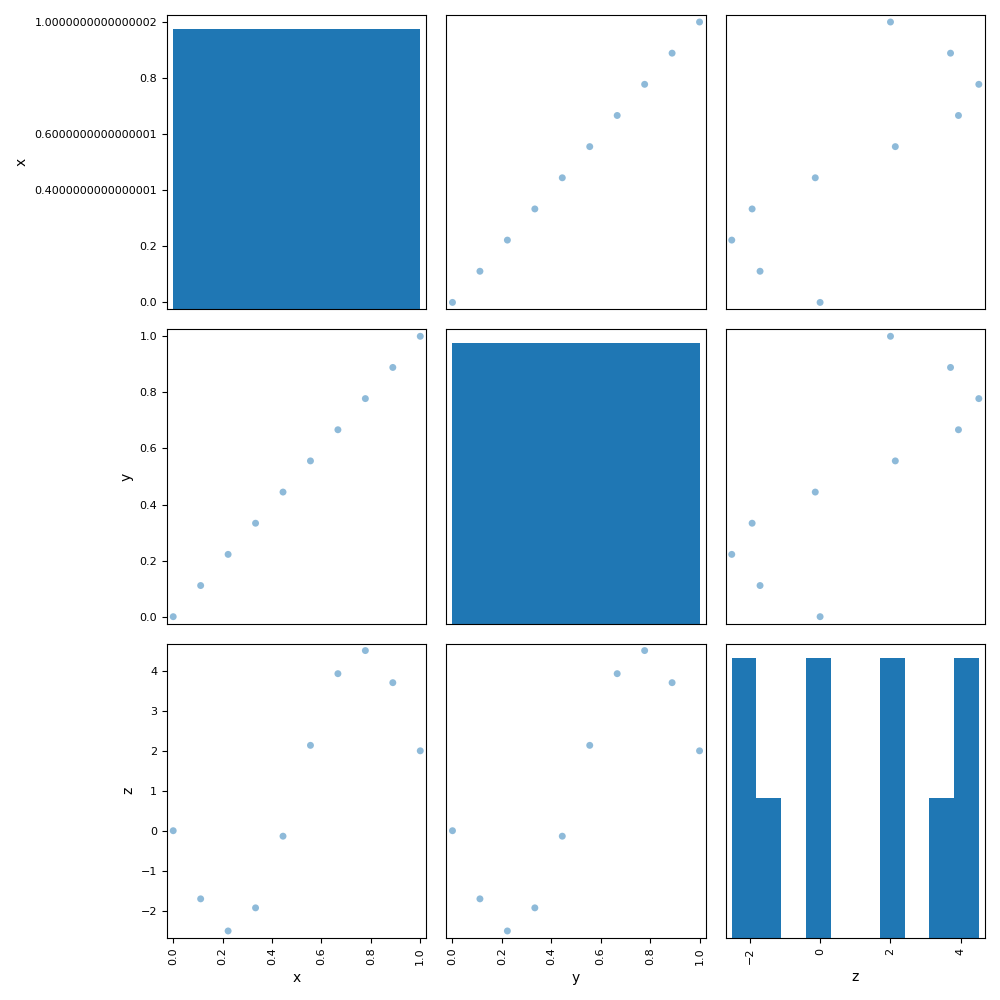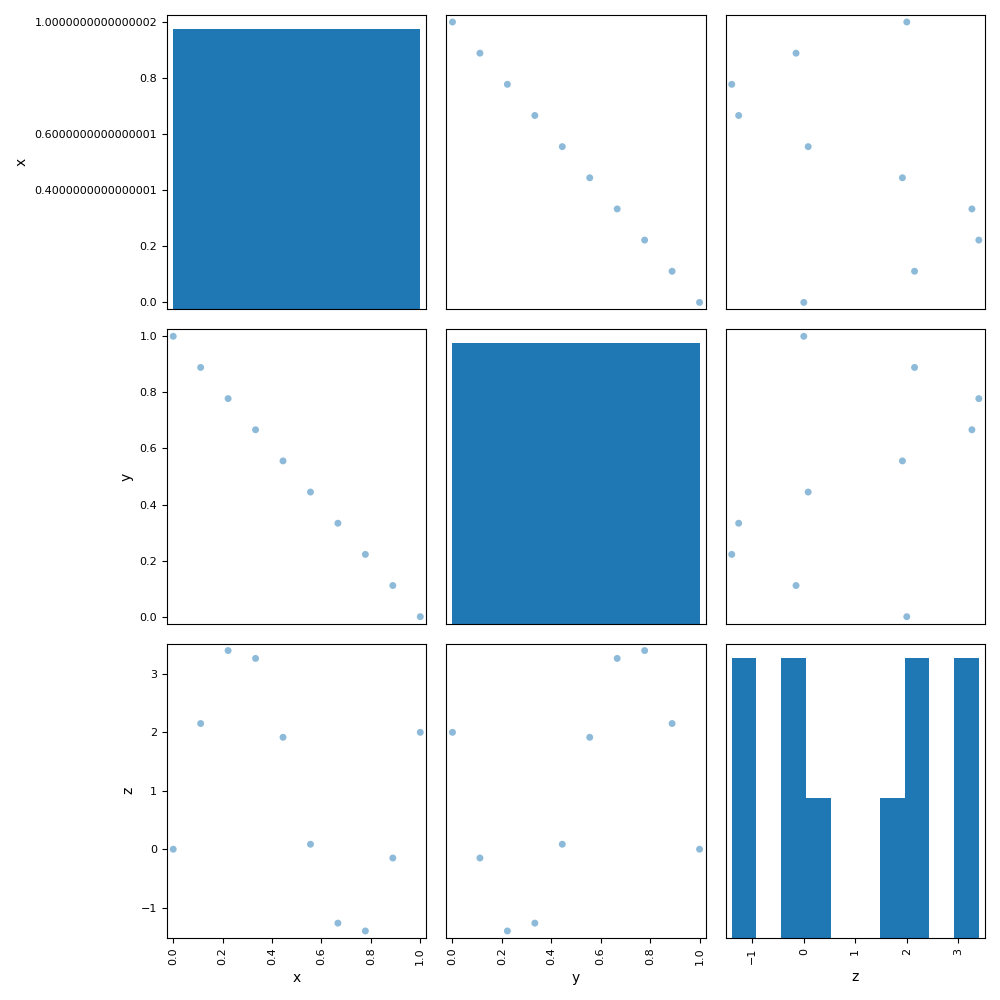# Diagonal design of experiments¶

Here is an illustration of the diagonal design of experiments (DOE) implemented by the DiagonalDOE class and used by the ScalableDiagonalModel. The idea is to sample the discipline by varying its inputs proportionally on one of the diagonals of its input space.

from __future__ import absolute_import, division, unicode_literals

from future import standard_library

from gemseo.api import (
configure_logger,
create_design_space,
create_discipline,
create_scenario,
)

configure_logger()

standard_library.install_aliases()


## Create the discipline¶

First, we create an AnalyticDiscipline implementing the function: $$f(x)=2x-3\sin(2\pi y)$$ and set its cache policy to "MemoryFullCache".

discipline = create_discipline(
"AnalyticDiscipline", expressions_dict={"z": "2*x-3*sin(2*pi*y)"}
)
discipline.set_cache_policy("MemoryFullCache")


## Create the design space¶

Then, we create a DesignSpace where $$x$$ and $$y$$ vary between 0 and 1.

design_space = create_design_space()


## Sample with the default mode¶

Lastly, we create a DOEScenario and execute it with the DiagonalDOE algorithm to get 10 evaluations of $$f$$. Note that we use the default configuration: all the disciplinary inputs vary proportionally from their lower bounds to their upper bounds.

scenario = create_scenario(
discipline, "DisciplinaryOpt", "z", design_space, scenario_type="DOE"
)
scenario.execute({"algo": "DiagonalDOE", "n_samples": 10})

dataset = discipline.cache.export_to_dataset()
dataset.plot("ScatterMatrix", save=False, show=True)Out:

<gemseo.post.dataset.scatter_plot_matrix.ScatterMatrix object at 0x7fc29e192b80>


## Sample with reverse mode for $$y$$¶

We can also change the configuration in order to select another diagonal of the input space, e.g. increasing $$x$$ and decreasing $$y$$. This configuration is illustrated in the new ScatterMatrix plot where the $$(x,y)$$ points follow the $$t\mapsto -t$$ line while the $$(x,y)$$ points follow the $$t\mapsto t$$ line with the default configuration.

discipline.cache.clear()
scenario = create_scenario(
discipline, "DisciplinaryOpt", "z", design_space, scenario_type="DOE"
)
scenario.execute(
{"algo": "DiagonalDOE", "n_samples": 10, "algo_options": {"reverse": ["y"]}}
)

dataset = discipline.cache.export_to_dataset()
dataset.plot("ScatterMatrix", save=False, show=True)Out:

<gemseo.post.dataset.scatter_plot_matrix.ScatterMatrix object at 0x7fc29e221250>


Total running time of the script: ( 0 minutes 1.278 seconds)

Gallery generated by Sphinx-Gallery